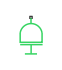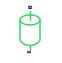# Inertia

Ideal mechanical rotational inertia

• Library:
• Simscape / Foundation Library / Mechanical / Rotational Elements

•## Description

The Inertia block represents an ideal mechanical translational inertia that is described with the following equation:

`$T=J\frac{d\omega }{dt}$`

where:

• T is inertia torque.

• J is inertia.

• ω is angular velocity.

• t is time.

By default, the block has one mechanical translational conserving port. The block positive direction is from its port to the reference point. This means that the inertia torque is positive if inertia is accelerated in the positive direction.

In some applications, it is customary to display inertia in series with other elements in the block diagram layout. To support this use case, the Number of graphical ports parameter lets you display a second port on the opposite side of the block icon. The two-port variant is purely graphical: the two ports have the same angular velocity, so the block functions exactly the same whether it has one or two ports. The block icon changes depending on the value of the Number of graphical ports parameter.

Number of graphical portsBlock Icon

`1``2`### Variables

To set the priority and initial target values for the block variables prior to simulation, use the Initial Targets section in the block dialog box or Property Inspector. For more information, see Set Priority and Initial Target for Block Variables.

Nominal values provide a way to specify the expected magnitude of a variable in a model. Using system scaling based on nominal values increases the simulation robustness. Nominal values can come from different sources, one of which is the Nominal Values section in the block dialog box or Property Inspector. For more information, see Modify Nominal Values for a Block Variable.

## Ports

### Conserving

expand all

Mechanical rotational conserving port that connects the inertia to the physical network.

Second mechanical rotational conserving port that lets you connect the inertia in series with other elements in the block diagram. This port is rigidly connected to port I, therefore the difference between the one-port and two-port block representations is purely graphical.

#### Dependencies

To enable this port, set the Number of graphical ports parameter to `2`.

## Parameters

expand all

Inertia value. The inertia is constant during simulation.

Select how to connect the block to the rest of the circuit:

• `1` — The block has one conserving port that connects it to the mechanical rotational circuit. When the block has one port, attach it to a connection line between two other blocks.

• `2` — Selecting this option exposes the second port, which lets you connect the block in series with other blocks in the circuit. The two ports are rigidly connected and have the same angular velocity, therefore the block functions exactly the same as if it had one port.

## Version History

Introduced in R2007a